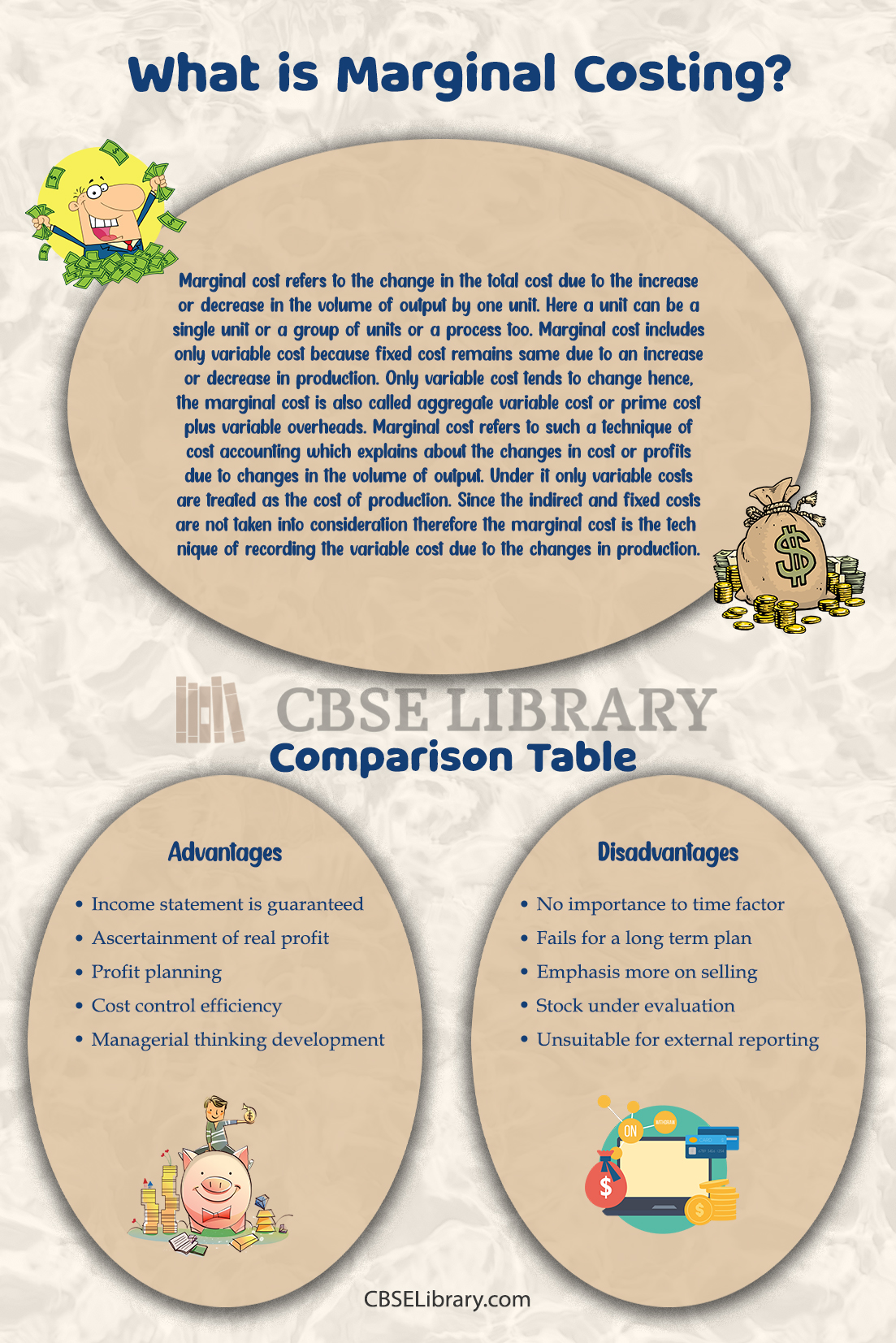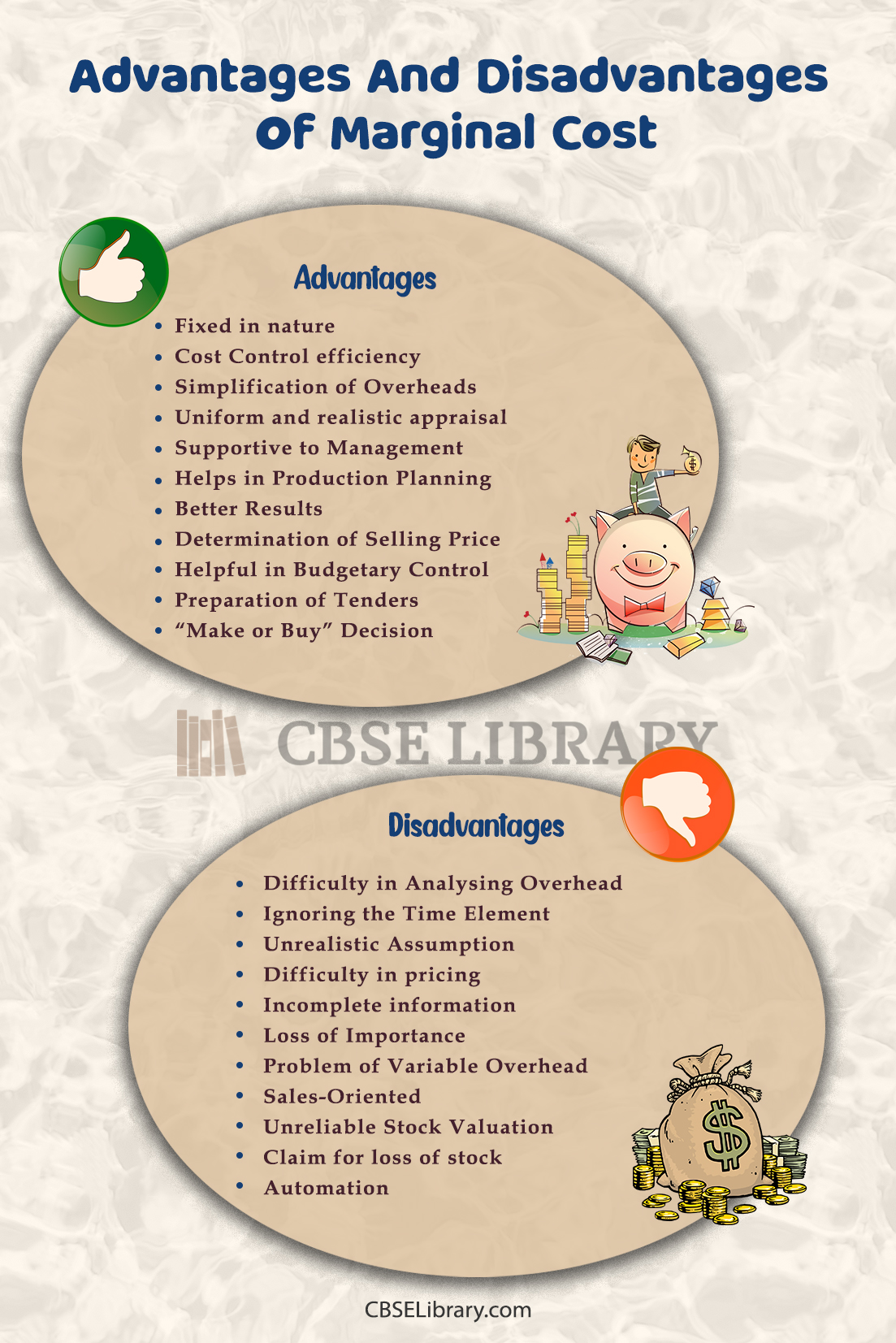# Advantages And Disadvantages Of Marginal Cost | What is Marginal Costing?, Pros and Cons, Formula And Characteristics

Advantages And Disadvantages of Marginal Cost: Marginal cost refers to the change in the total cost due to the increase or decrease in the volume of output by one unit. Here a unit can be a single unit or a group of units or a process too. Marginal cost includes only variable cost because fixed cost remains same due to an increase or decrease in production. Only variable cost tends to change hence, the marginal cost is also called aggregate variable cost or prime cost plus variable overheads. Marginal cost refers to such a technique of cost accounting which explains about the changes in cost or profits due to changes in the volume of output. Under it only variable costs are treated as the cost of production. Since the indirect and fixed costs are not taken into consideration therefore the marginal cost is the technique of recording the variable cost due to the changes in production.

Students can also find more Advantages and Disadvantages articles on events, persons, sports, technology, and many more.

## What is a Marginal Cost? What are the Advantages and Disadvantages of Marginal Cost?

Marginal cost refers to the change in the total cost due to the increase or decrease in the volume of output by one unit. There are several characteristics of marginal cost-

1. Techniques of cost analysis: marginal cost is not the traditional method of costing. Rather it is a technique that deals with the effect of change in cost, volume, price etc.
2. Division of costs: all the elements of the cost production, which is production, selling, distribution, are divided into variable and fixed costs.
3. Periodic cost and product cost: marginal cost clarifies the difference between periodic cost and product cost. Fixed cost is termed as period cost as it is related to a specific period of time. Variable cost or marginal cost is termed as the product cost.
4. Calculations of profits: here, the profits can be determined in a proper manner. Under it, contribution is calculated by deducting the marginal cost from total sales and net profit is ascertained by deducting the fixed cost from the contribution.

### Calculating Marginal Cost

Calculating marginal cost helps the business determine the point at which increasing the number of goods produced will increase average cost. Costs can add up if the company needs to add equipment, move to a larger facility, or struggle to find a supplier who provides enough materials. Formula to calculate the marginal cost follows as-

Marginal cost=(change in cost) /(change in quantity)  MC=ΔCΔQ

MC= Marginal Cost

{ΔC}=change in cost

{ΔQ}=change in quantity

When charted on a graph, marginal cost follows a U shape. Unless the fixed cost is covered, the cost starts getting higher. It stays at that low point for a period, and then begins to creep in as the increased production requires spending money for more employees, equipment, etc. Understanding the marginal cost of a product helps a company to assess its profitability and make informed decisions related to the product, including pricing.Fixed in nature: Variable cost fluctuates from time to time, but in the long run, marginal cost is constant. Marginal cost remains the same regardless of the volume of production.

Cost Control efficiency: It fractionates the cost into fixed and variable. Fixed costs are excluded from the product. As such, management controls marginal cost-effectively.

Simplification of Overheads: It reduces the degree of over- or under-recovery of overheads due to separation of fixed overheads from production cost.

Uniform and realistic appraisal: As fixed overhead costs are excluded from product costing, evaluation of work-in-progress and finished goods becomes more realistic.

Supportive to Management: It enables the management to start a new line of production which is profitable. This is helpful in determining which product is profitable to buy or manufacture. Management can take decisions regarding pricing and tendering.

Helps in Production Planning: It shows the amount of profit at every stage of production with the help of cost quantity profit relation. Here a break-even chart is used.

Better Results: If marginal cost is used with standard costing, it gives even better results.

Determination of Selling Price: The difference between fixed cost and variable cost is very helpful in determining the selling price of products or services. Sometimes, different prices are charged for the same commodity in different markets to meet different degrees of competition.

Helpful in Budgetary Control: Budgeting for different levels and categorization of expenses into flexible budgets is very helpful.

Preparation of Tenders: Many business enterprises have to compete in the market to quote the lowest price. The total variable cost, when calculated separately, becomes the ‘floor price’. Any price above this minimum price may be quoted to increase the total contribution.

Difficulty in Analysing Overhead: Separating costs into fixed and variable is a difficult problem. In marginal cost, both fixed and variable cost are not considered.

Ignoring the Time Element: Fixed Cost and Variable Cost differ in the short run; But in the long run, all costs are variable. All costs change in the long run at different levels of operation. When new plant and equipment are introduced, fixed costs and variable costs will differ.

Unrealistic Assumption: The selling price assumption will remain the same at different levels of operation. In real life, they can change and give unrealistic results.

Difficulty in pricing: The selling price under marginal cost is determined on the basis of contribution. It is very difficult to fix the price in case of cost plus contract.

Incomplete information: It does not give a reason for increase in production or sales.

Loss of Importance: In capital-intensive industries, fixed costs occupy a major part of the total cost. But marginal cost only covers variable costs. As such, it loses its importance in capital industries.

Problem of Variable Overhead: Marginal Costing removes the problem of over and under absorption of fixed overheads. Yet there is a problem in terms of variable overheads.

Sales-Oriented: Successful business has to go in a balanced way with respect to selling production functions. But marginal costing is criticised for giving more importance to its selling function. Thus it is called sales-oriented. Less importance is given to the production function.

Unreliable Stock Valuation: Under marginal cost stock of work-in-progress and ready stock is valued at variable cost only. No part of the fixed cost is added to the value of the stock.

Claim for loss of stock: An insurance claim for loss or damage to the stock based on such valuation would be prejudicial to the business.

Automation: Nowadays increasing automation is leading to an increase in fixed costs. If such rising fixed costs are neglected, the cost-fixing system cannot be effective and reliable.

 Advantages of marginal cost Disadvantages of marginal cost Income statement is guaranteed No importance to time factor Ascertainment of real profit Fails for a long term plan Profit planning Emphasis more on selling Cost control efficiency Stock under evaluation Managerial thinking developmentQuestion 1.
What is the marginal cost depended on?

It depends on the total volume of the goods being produced.

Question 2.
How can marginal cost be increased?